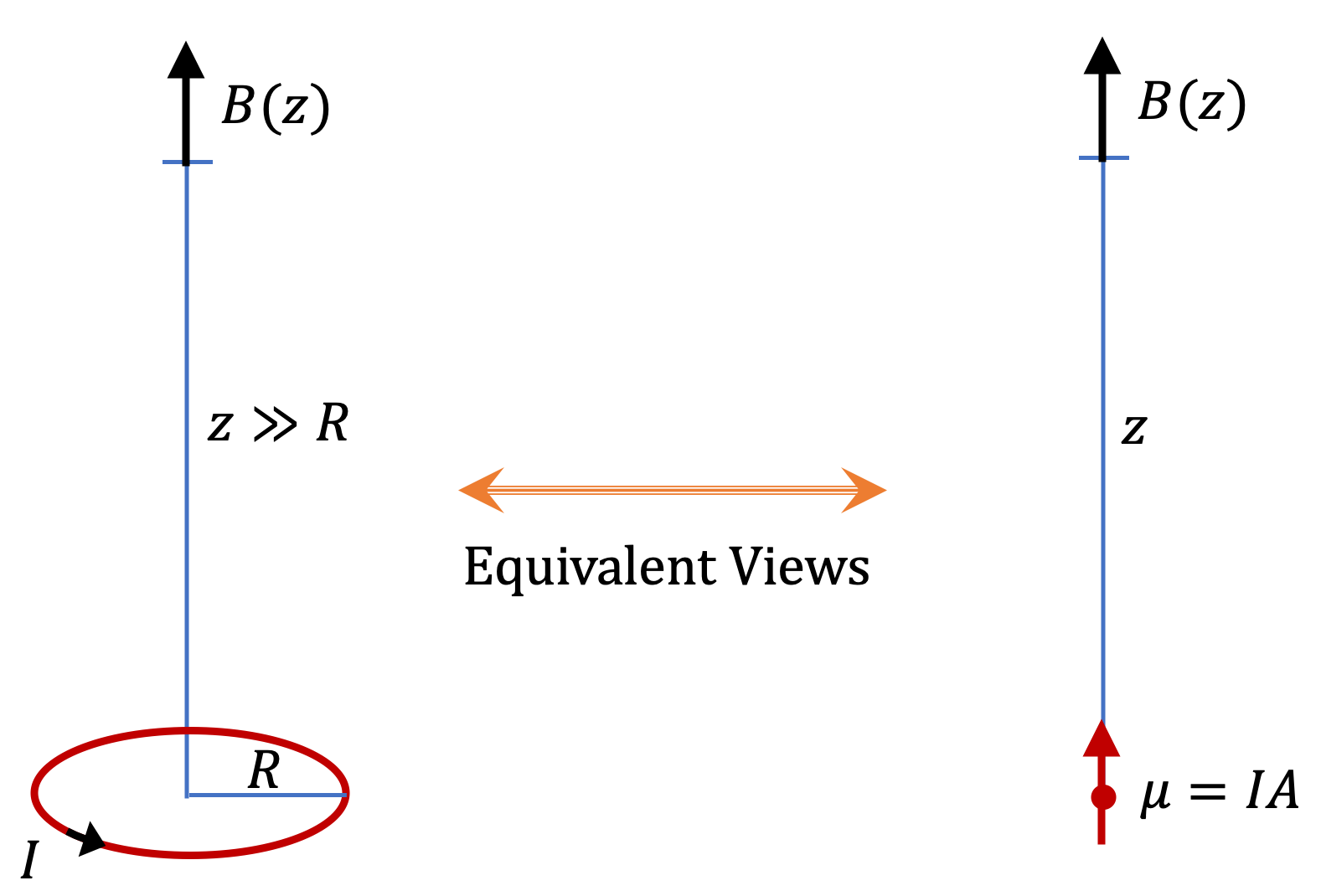## Section39.2Magnetic Dipole Field

The magnetic field of a current in a circular loop given in Eq. (39.1.9) has interesting limiting behaviors. We will now show that for microscopic loops, magnetic field varies as inverse cube of the distance from the loop. This is similar to the way electric field of an electric dipole varies. Therefore, we will interpret this type of magnetic field as magnetic field of an ideal magnetic dipole, i.e., a microscopic magnet.

To obtain the distance-dependence of a micoscopic loop we look at Eq. (39.1.9) for $z \gg R$ and find the leading behavior using Maclauren expansion using variable $\epsilon = R/z \text{.}$ This will give us magnetic field in direction perpendicular to the loop.

\begin{align*} B_P \amp = \frac{\mu_0I_R^2}{2} \lim_{z \gg R} \dfrac{1}{\left(R^2 + z^2\right)^{3/2}} = \frac{\mu_0I_R^2}{2z^3} \lim_{\epsilon \ll 0} \dfrac{1}{\left(1 + \epsilon^2\right)^{3/2}}, \\ \amp = \frac{\mu_0I_R^2}{2z^3} \lim_{\epsilon \ll 0} \left[ 1 - \frac{3}{2}\,\epsilon^2 + \cdots\right] = \frac{\mu_0I_R^2}{2} \frac{1}{z^3}. \end{align*}

Introducing factors of $\pi$ and rewriting the field in the following way helps with the identification of the magnetic dipole moment of the current loop in the formula, which we will denote by symbol $\mu\text{,}$ as before. This field is called dipole field and denoted by attaching a subscript to the symbol for the field.

\begin{align*} B_{\textrm{dipole}} \amp = \frac{\mu_0}{2\pi} \frac{I\pi R^2}{z^3} = \frac{\mu_0}{2\pi} \frac{IA}{z^3} = \frac{\mu_0}{2\pi} \frac{\mu}{z^3} \end{align*}

The $z \gg R$ limit on the field of a current loop provides two views of magnetic phenomenon - as tiny current loops and as magnetic dipole as illustrated in Figure 39.2.1.Figure 39.2.1. Two views of small current loop. Magnetic field on the axis of a current loop when field point is far away, i.e., when $z \gt\gt R\text{,}$ $B\sim 1/z^3\text{,}$ which is the same as magnetic field of a dipole of magnetic dipole moment, $\mu=IA\text{,}$ with $A$ the area of the loop.